# ML Wiki

## Extensible Hashing

Hash-based secondary memory index structure for databases

Main ideas:

• Growing hash function
• Directory

### Growing Hash Function

Variables we use:

• $b$ - length of bit-string that Hash Function outputs (typically 64)
• $i$ - number of bits we can use
• as number of keys grows, we increase $i$
•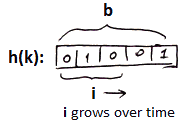### Directory

Directory introduces additional level of indirection

•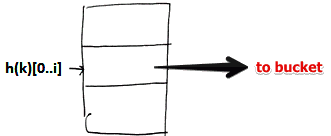• here we keep all possible combinations of $i$ bits with pointers to associated buckets

Example

• suppose for key $k$: $h(k) = \fbox{1010}$
• we take first $i$ bits of this value, i.e. $h(k)[0..i] = 1$
• so we find record with 1 in the directory and this way we locate the needed bucket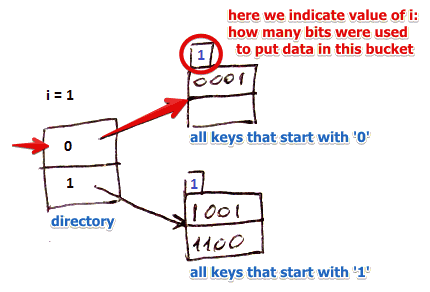## Operations

### Lookup

• we take first $i$ bits of hash and find a corresponding record in the directory
• then we follow the pointer and find the whole key (all $b$ bits) there

### Insert

#### Simple Case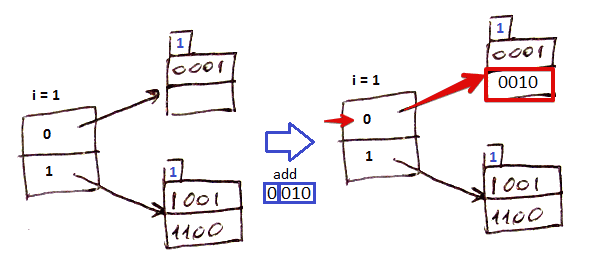• suppose $i = 1$
• for key $k:$ $h(k) = 0010$ and first $i$ bits are 0: look it up in the directory
• there is enough room so add in there

#### Creating New Directory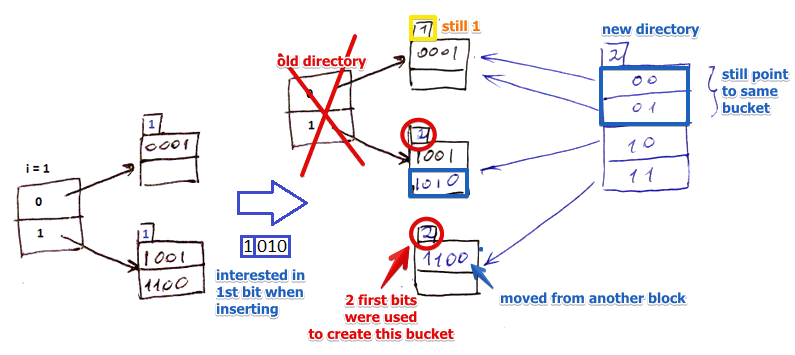• suppose $i = 1$
• given hash $h(k) = 1010$ we extract first 1 bit which is 1
• we look 1 up in the directory and follow the pointer
• but there's no room in this block
• so we split it into 2 parts
• that means that both blocks use 2 bits to assign a key to a bucket (and we indicate that value on top of the buckets)
• but to address these new buckets now we need 2 bits, and still $i = 1$
• i.e. $i$ in the directory becomes less than at least one $i$ from buckets
• that means we need to create a new directory
• if it wasn't the case, we just would re-wire pointers to the dict
• note that bucket for (0) is still the same - so both 00 and 01 in the new directory point to this bucket
• if now we insert 0000 and 0100, we will reorganize the first bucket, but will not rebuild the directory

So the rule is:

• if $i$ for one of the bucket grows more than $i$ of the directory
• we need to rebuild the prefix directory
• all other blocks are kept untouched (otherwise we would have to re-organize the whole thing)

### Deletion

Just the opposite of #Insertion

## Summary

Pros

Cons

• another level of indirection. Not that bad if we can store the entire directory in memory, but it's not often the case
• size of directory growth is exponential to $i$ - quickly becomes the bottleneck and may introduce a lot of latency
• it fits into memory and then all of a sudden it doesn't

Linear Hashing is another alternative that can handle growing files better